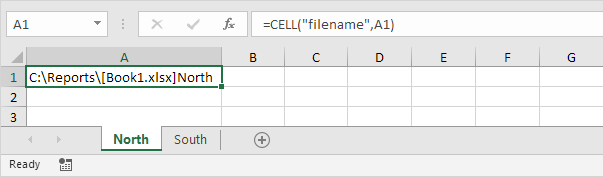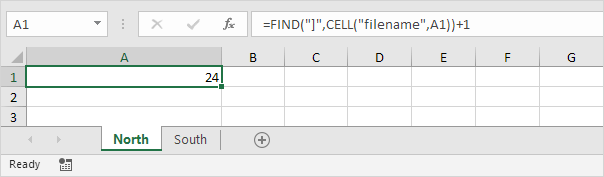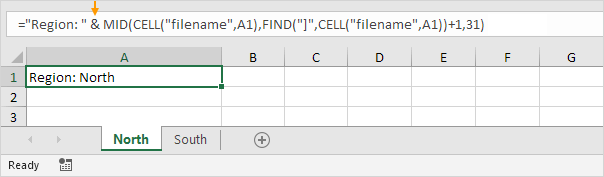# Get Sheet Name

To return the sheet name in a cell, use CELL, FIND and MID in Excel. There's no built-in function in Excel that can get the sheet name.

1. The CELL function below returns the complete path, workbook name and current worksheet name.Note: instead of using A1, you can refer to any cell on the first worksheet to get the name of this worksheet.

2. Use the FIND function to find the position of the right bracket. Add 1 to return the start position of the sheet name.3. To extract a substring, starting in the middle of a string, use the MID function. First argument (formula from step 1). Second argument (formula from step 2). Third argument (31).Explanation: the MID function shown above starts at position 24 and extracts 31 characters (maximum length of a worksheet name).

4. You may want to add text to the name of the sheet. Simply use the ampersand (&) operator as shown below.5. To get the name of the second worksheet, simply refer to any cell on the second worksheet.Pro tip: use Excel VBA to display the sheet names of all Excel files in a directory. You can find detailed instructions here.

Go to Next Chapter: Format Cells